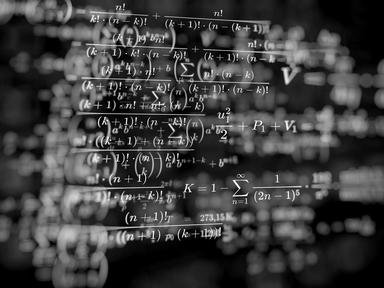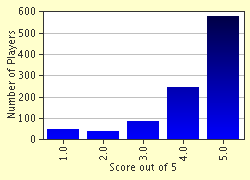FREE! Click here to Join FunTrivia. Thousands of games, quizzes, and lots more!# Small Derivatives Quiz

### This is an easy quiz for anyone who took calculus. Good Luck!

A multiple-choice quiz by Lanire. Estimated time: 3 mins.

Author
Lanire
Time
3 mins
Type
Multiple Choice
Quiz #
69,815
Updated
Dec 03 21
# Qns
5
Difficulty
Average
Avg Score
4 / 5
Plays
8306
Last 3 plays: Guest 73 (3/5), Guest 175 (5/5), Guest 75 (4/5).
This quiz has 2 formats: you can play it as a or as shown below.
Scroll down to the bottom for the answer key.
1. What is the derivative of 'y = 3x squared'? Hint

5x squared
5x
6x
6x squared

#### NEXT>

2. What is the derivative of 'f(y) = 2y to the power of one third'? Hint

seven thirds y to the power of seven thirds
two thirds y to the power of negative two thirds
two thirds y to the power of two thirds
seven thirds y to the power of negative seven thirds

#### NEXT>

3. What is the derivative of 'y = 2x squared - 3x + 4'? Hint

4x squared
4x squared - 3x
4x - 3
4x squared - 3x + 1

#### NEXT>

4. What is the derivative of 'y = (2x + 3)squared'? Hint

4 (2x + 3)
2 (2x + 3) squared
2 (2x + 3)
4 (2x + 3) squared

#### NEXT>

5. What is the derivative of 'f(x) = x(x + 1)'? Hint

x + 1
2x squared + 1
2x + 1
2x squared + x

 (Optional) Create a Free FunTrivia ID to save the points you are about to earn:Select a User ID:Choose a Password:Your Email:

Quiz Answer Key and Fun Facts
1. What is the derivative of 'y = 3x squared'?

The example illustrates the Power Rule for derivatives. Using the rule, you multiply the power of x by the constant before x and reduce the power of x by one to get the derivative.
2. What is the derivative of 'f(y) = 2y to the power of one third'?

Answer: two thirds y to the power of negative two thirds

The Power Rule as in the first question: multiply the power of x by the constant before x and reduce the power of x by one to get the derivative.
3. What is the derivative of 'y = 2x squared - 3x + 4'?

This derivative can be found using the Sum Rule.
4. What is the derivative of 'y = (2x + 3)squared'?

This is an example of how a Chain Rule is used. Firstly, you take the power of the bracket and multiply it by the bracket itself taking one power off the bracket. Then you take the derivative of the expression inside the bracket and multiply it by the number in front of the bracket.
5. What is the derivative of 'f(x) = x(x + 1)'?

This can be solved using either the Product Rule or by multiplying x through the bracket and using the Sum Rule.
Source: Author Lanire

This quiz was reviewed by FunTrivia editor rossian before going online.
Any errors found in FunTrivia content are routinely corrected through our feedback system.
Most Recent Scores
Jun 01 2023 : Guest 73: 3/5
May 27 2023 : Guest 175: 5/5
May 27 2023 : Guest 75: 4/5
May 26 2023 : emmal2000uk: 1/5
May 26 2023 : Guest 49: 3/5
May 20 2023 : Guest 175: 5/5
May 17 2023 : Guest 121: 4/5
May 16 2023 : Guest 86: 4/5
May 16 2023 : Guest 64: 5/5

Score Distribution